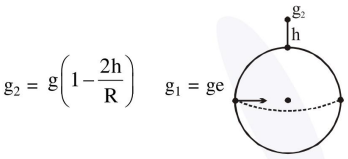# The acceleration due to gravity on the earth's surface

Question:

The acceleration due to gravity on the earth's surface at the poles is $\mathrm{g}$ and angular velocity of the earth about the axis passing through the pole is $\omega$. An object is weighed at the equator and at a height $\mathrm{h}$ above the poles by using a spring balance. If the weights are found to be same, then $h$ is : ( $h< 1.$\frac{\mathrm{R}^{2} \omega^{2}}{8 \mathrm{~g}}$2.$\frac{\mathrm{R}^{2} \omega^{2}}{4 \mathrm{~g}}$3.$\frac{R^{2} \omega^{2}}{g}$4.$\frac{\mathrm{R}^{2} \omega^{2}}{2 \mathrm{~g}}$Correct Option: , 4 Solution:$g_{e}=g-R \omega^{2}$$\mathrm{g}_{2}=\mathrm{g}-\frac{2 \mathrm{gh}}{\mathrm{R}}$Now$R \omega^{2}=\frac{2 g h}{R}\mathrm{h}=\frac{\mathrm{R}^{2} \omega^{2}}{2 \mathrm{~g}}\$